# The TickRate UGen

I’ve received several questions about the TickRate UGen that I’ve used in some recent Sound Bytes. Quite simply, this is a UGen that allows you specify an audio generation rate. Setting a rate of 1 means that the UGen patched to TickRate will be ticked every time that TickRate is. Setting a rate of 0.5 means that the UGen patched to TickRate will be ticked at half the rate of TickRate (every other sample). Setting a rate of 2 means that the UGen patched to TickRate will be ticked twice every time that TickRate is ticked. The sample frame that TickRate generates can either be the same as the most recently generated sample frame from the UGen patched to it, or you can have it interpolate between that sample frame and the next sample frame from the UGen patched to it. At low tick rates, non-interpolated audio will sound like it is being bit-crushed. Essentially, this UGen allows you to control the sample rate of any UGen patched to it, with the limitation that you won’t be able to patch the output of that UGen anywhere else. That limitation is not enforced, but if you do so, your audio will not sound correct.

As for where to get the TickRate UGen, you can either copy the file from the Minim GitHub repo, or you can pull the repo and build the library locally.

# Sound Byte: Glitch Generator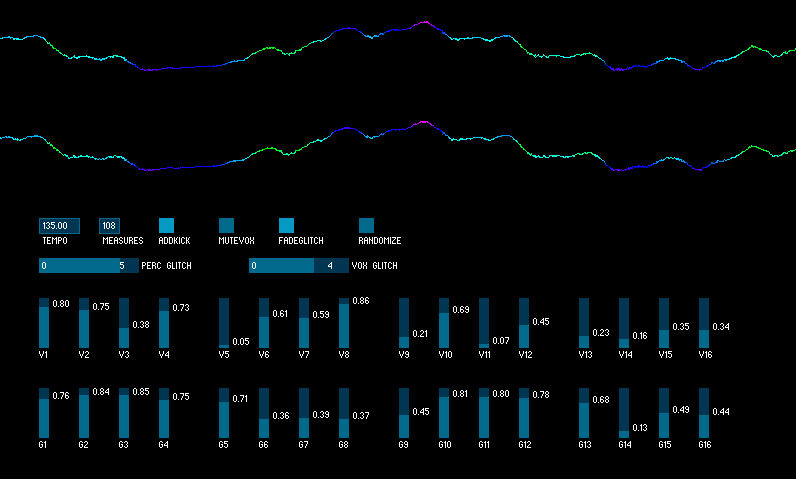I’ve been working on this one for a few days. It follows the same principle as the beat generator from my previous post: whether or not a note is added to the generated sequence at a given step is determined by a probability. However, unlike the beat generator, this one doesn’t sequence distinct sounds. Instead, it is essentially generating timed control changes for effects on two sound files that are continually playing.

The first file is the vocal track from Half Life by Imogen Heap. Its playback rate is adjusted to the chosen tempo and “notes” in the sequence for it are turning on a sample-repeat effect. For each trigger of the effect, the length of the sampled audio is determined randomly based on the settings in the Vox Glitch range.

The second file is a loop from the beginning of Hydra Remix By Koen Groeneveld. The notes in the sequence for that track are setting loop points in a looping FilePlayer, though the resulting sound is the exact same kind of thing going on with the vocals. Once again, the length of the repeated audio is determined based on the settings in the Perc Glitch range. You can also specify whether you want each triggered glitch to fade in over its duration or not, which is kind of a nice effect.

Finally, you can choose to have a steady kick drum play underneath all the glitching to give yourself a good reference point. I’ve had a lot of fun playing with different settings, it’s like endless minimal, glitchy remixes of Imogen Heap. Try it out!

# Sound Byte: Beat Generator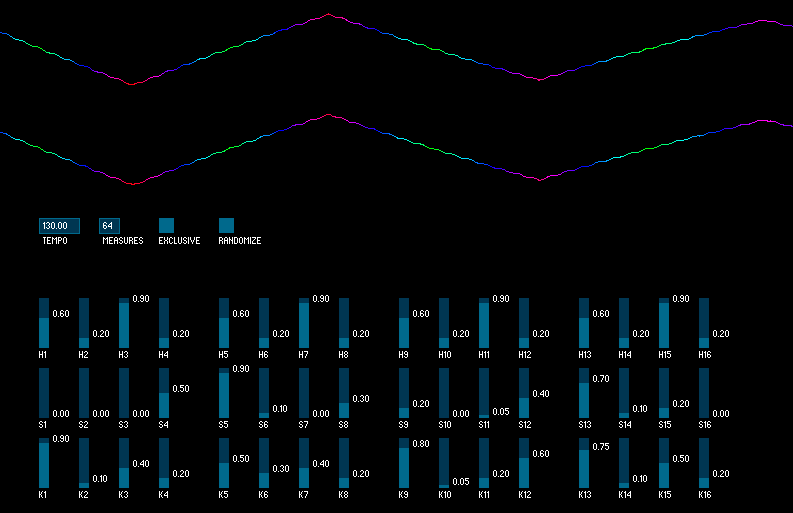Probably the coolest Sound Byte yet, this one will generate drum beats based on probabilities for each sixteenth note in a measure. The sound-set is basic: kick, snare, hat, plus a chime loop that is overlaid for a little melodic flavor. You can tweak the probability of all the steps independently, or just click a button to set them all to new random values. Two “modes” allow you to specify whether you want the kick and snare to ever play at the same time and some simple rules are used to determine the amplitude of each note. I considered putting in panning of some kind, but decided it could go in a later iteration. I may do a bassline generator along the same lines at some point. Check it out!

# Sound Byte: Broken Record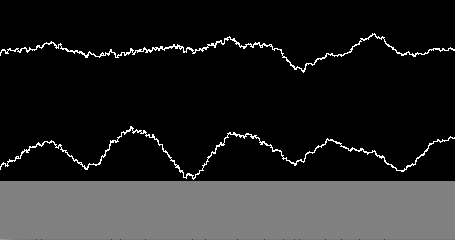I wrote a UGen the other day that allows you to change the tick rate (or sample rate, if you like), of any UGen that you patch to it. After making a mouse-driven example to test it out, I created this example so that the change in the tick rate could be driven by the audio itself. Here, the current loudness (RMS amplitude, to be exact) of the audio being looped directly adjusts the playback speed. Louder parts result in a higher tick rate. What you get sounds like a really broken record player. Not only is it stuck looping the same four bars, but it sounds like the timing belt is having all sorts of issues. Check it out!

# Sound Byte: Filtered Tuning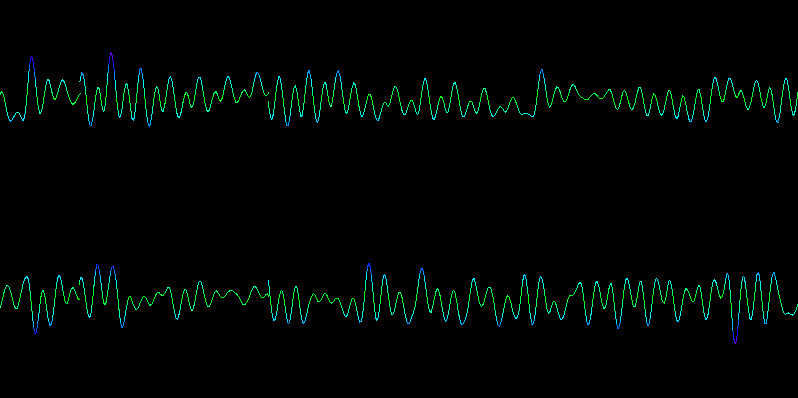I’ve been meaning to try out some digital Moog filter code. A digital Moog filter is simply a filter that attempts to model the analog filters found on Moog synthesizers. It’s got two knobs: frequency and resonance. I yanked some code from musicdsp.org, a wonderful resource, and wrote a really simple UGen with it. I meant to write more of a song, but there’s never enough time for that in these evening coding forays. So instead, you get this kind of avant-garde slow tuning thing using amplitude modulation and tweaking of the filter values. Have a listen!

# Sound Bytes: Oscil as a looping sampler

Eventually, we plan to add a UGen to Minim that will allow you to control the generating speed of any other UGen, but that got me thinking about how you might do something similar with existing components. So, I struck upon the idea of using an Oscil as a looping sampler by using an audio file as the Waveform and setting the frequency of the Oscil to be very low. The fun thing about this is that if you set the Oscil to a negative frequency, the sound will play in reverse. Additionally, I thought it’d be fun to be able to automate the changing of the frequency by having an LFO control the frequency of each Oscil (left and right channels of the original audio file). So here’s a sketch that lets you play with this setup: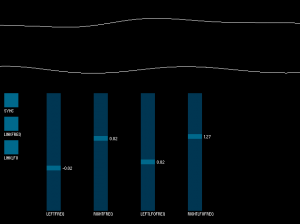# Sound Byte: Mass-Spring-Damper System

I’m picking my way through Real Sound Synthesis for Interactive Applications by Perry R. Cook (one of the authors of the STK) and decided to start making small apps to demonstrate the different kinds of synthesis he covers in the book. This first one let’s you set the variables of a mass-spring-damper system and then trigger it, which is exactly the same as setting the coefficients of a two-pole IIR filter and sending an impulse into it (as it turns out). Check it:# Frequency Modulation Is Easy

I’m working on adding the ability to do frequency modulation in Minim. I didn’t figure it was a very complicated thing. I knew that I’d have to do it while generating each sample, so it couldn’t be done as an `AudioEffect`. But when I went looking online about how to do it, I found a number of articles that described the technique in words, with somewhat dense equations, but none that had easy to understand code examples. I did find this article, but the code in it is some form of Lisp, a language I am not familiar with. I thought I had it figured out a couple times, but every time I tried something new I still wasn’t achieving the correct result.

So today I asked my friend The Mysterious H how it works because he’s a smart guy and builds synths out of Atari sound chips and shit like that. He goes, “Oh it’s easy.”

Every time you want to generate a sample of the signal you are modulating (the carrier), generate a sample of your modulator and add that to the phase you use to generate your carrier sample.

Said another way: Say you’ve got a signal called f. At every time step t (which is your phase), you generate a sample s by evaluating f(t). If you want to modulate the frequency of f with a modulator called m, then at every t you will do this:

The speed of the modulation is determined by the frequency of your modulator and the amount of the modulation (how many hertz above and below the frequency of your carrier signal the audible signal will swing) is determined by the amplitude of the modulator. In my tests I found that the amplitude had to be around 0.001 to achieve what one would consider vibrato. Setting it to 0.1 made for some pretty intense alien sounds when the frequency of the modulator was up in audio signal range (20 Hz and up, say).

It’s lots of fun to play with and will be coming soon!

# Minim: An Audio Library for Processing

It’s here, the first release of my audio library for Processing: Minim.

Here are some of the cool features:

• Released under the GPL, source is included in the full distribution.
• AudioFileIn: Mono and Stereo playback of WAV, AIFF, AU, SND, and MP3 files.
• AudioFileOut: Mono and Stereo audio recording either buffered or direct to disk.
• AudioInput: Mono and Stereo input monitoring.
• AudioOutput: Mono and Stereo sound synthesis.
• AudioSignal: A simple interface for writing your own sound synthesis classes.
• Comes with all the standard waveforms, a pink noise generator and a white noise generator. Additionally, you can extend the Oscillator class for easy implementation of your own periodic waveform.
• AudioEffect: A simple interface for writing your own audio effects.
• Comes with low pass, high pass, band pass, and notch filters. Additionally, you can extend the IIRFilter class for easy implementation of your own IIR filters.
• Easy to attach signals and effects to AudioInputs and AudioOutputs. All the mixing and processing is taken care of for you.
• Provides an FFT class for doing spectrum analysis.
• Provides a BeatDetect class for doing beat detection.

Visit the download page, then take a look at the Quickstart Guide or dive right into the Javadocs.

# FFT Averages

This post assumes you already know what an FFT is, so if you don’t, I suggest reading Chapter 8 and Chapter 12 of The Scientist and Engineer’s Guide to Digital Signal Processing. What I’m going to discuss is two different ways of averaging contiguous bands of an FFT. Before I do that, I’d like to review exactly what the frequency bands of an FFT represent.

What’s In An FFT?

Let’s say you have a sample buffer that has 1024 samples in it. If you run this through an FFT you will get a frequency domain described by two arrays that are each 1024 values long. However, typically the values in these arrays are not used directly. Instead, each pair of values (`real` and `imag`, `real` and `imag`, etc) is used to calculate the amplitude of each point in the FFT and that value is then used as the value of the spectrum at that point. Even more confusing to those new to the FFT is that the spectrum of 1024 samples is only 513 values long (the array runs from 0 to 512). The reason for this is because the values above `spectrum` are, in practice, meaningless because they represent frequencies above the Nyquist frequency (one-half the sampling rate). So now we’ve simplified our two 1024 value arrays down to one array that is 513 values long. What do these 513 values represent, exactly?

Each point of the FFT describes the spectral density of a frequency band centered on a frequency that is a fraction of the sampling rate. Spectral density describes how much signal (amplitude) is present per unit of bandwidth. In other words, each point of an FFT is not describing a single frequency, but a frequency band whose size is proportional to the number of points in the FFT. The bandwidth of each band is 2/N, expressed as a fraction of the total bandwidth (i.e. N/2, which corresponds to the point at one-half the sampling rate), where N is the length of the time domain signal (1024 in our example). The exceptions to this are the first and last bands (`spectrum` and `spectrum`), whose bandwidth is 1/N. This makes more sense when talking about actual frequencies, so:

Given a sample buffer of 1024 samples that were sampled at 44100 Hz, a 1024 point FFT will give us a frequency spectrum of 513 points, with a total bandwidth of 22050 Hz. Each point i in the FFT represents a frequency band centered on the frequency i/1024 * 44100 whose bandwidth is 2/1024 * 22050 = 43.0664062 Hz, with the exception of `spectrum` and `spectrum`, whose bandwidth is 1/1024 * 22050 = 21.5332031 Hz. Knowing this, we can get on to the business of averaging contiguous bands.

Linear Averages

We’ve got an FFT with 513 spectrum values, but we want to represent the spectrum as 32 bands, so we’ve decided to simply group together frequency bands by averaging their spectrum values. The obvious way to do this is to simply break the 513 values into 32 chunks of (nearly) equal size:

```int avgWidth = (int)513/32;
for (int i = 0; i < 32; i++)
{
float avg = 0;
int j;
for (j = 0; j < avgWidth; j++)
{
int offset = j + i*avgWidth;
if ( offset < 513 )
{
avg += spectrum[offset];
}
else break;
}
avg /= j;
averages[i] = avg;
}```

The problem with this method is that most of the useful information in the spectrum is all below 15000 Hz. By creating average bands in a linear fashion, important detail is lost in the lower frequencies. Consider just the first average band in this example: it corresponds roughly to the frequency range of 0 to 689 Hz, that’s more than half of the keyboard of a piano!

Logarithmic Averages

A better way to group the spectrum would be in a logarithmic fashion. A natural way to do this is for each average to span an octave. Starting from the top of the spectrum, we could group frequencies like so (this assumes a sampling rate of 44100 Hz):

11025 to 22050 Hz
5512 to 11025 Hz
2756 to 5512 Hz
1378 to 2756 Hz
689 to 1378 Hz
344 to 689 Hz
172 to 344 Hz
86 to 172 Hz
43 to 86 Hz
22 to 43 Hz
11 to 22 Hz
0 to 11 Hz

This gives us only 12 bands, but already it is more useful than the 32 linear bands. We could now easily track a kick drum and snare drum, for example. If we want more than 12 bands, we could split each octave in two, or three, or whatever, the fineness would be limited only by the size of the FFT.

Knowing what frequency each point in the FFT corresponds to, and also how wide the frequency band for that point is, allows us to compute the logarithmically spaced averages. First we need to be able to map a frequency to the FFT spectrum. These functions will accomplish that (`timeSize` is N):

```public float getBandWidth()
{
return (2f/(float)timeSize) * (sampleRate / 2f);
}

public int freqToIndex(int freq)
{
// special case: freq is lower than the bandwidth of spectrum
if ( freq < getBandWidth()/2 ) return 0;
// special case: freq is within the bandwidth of spectrum
if ( freq > sampleRate/2 - getBandWidth()/2 ) return 512;
// all other cases
float fraction = (float)freq/(float) sampleRate;
int i = Math.round(timeSize * fraction);
return i;
}```

This may not seem clear at first, but it is simply the inverse of mapping an index to a frequency, which was mentioned above: Freq(i) = (i/timeSize) * sampleRate. Hereâ€™s how weâ€™d use these functions to compute the logarithmic averages listed above:

```for (int i = 0; i < 12; i++)
{
float avg = 0;
int lowFreq;
if ( i == 0 )
lowFreq = 0;
else
lowFreq = (int)((sampleRate/2) / (float)Math.pow(2, 12 - i));
int hiFreq = (int)((sampleRate/2) / (float)Math.pow(2, 11 - i));
int lowBound = freqToIndex(lowFreq);
int hiBound = freqToIndex(hiFreq);
for (int j = lowBound; j <= hiBound; j++)
{
avg += spectrum[j];
}
// line has been changed since discussion in the comments
// avg /= (hiBound - lowBound);
avg /= (hiBound - lowBound + 1);
averages[i] = avg;
}```

This is hard coded to compute only 12 averages, which is not ideal, but it would be easy enough to determine the number of octaves based on the sample rate and the smallest bandwidth desired for a single octave.

LinLogAverages is an applet demonstrating the difference between linear averages and logarithmic averages.